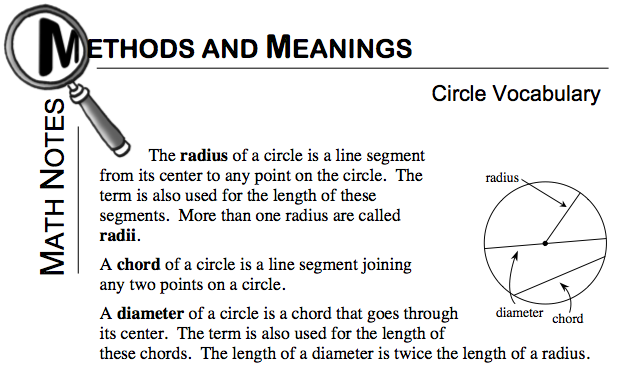Home > ACC7 > Chapter cc28 > Lesson cc28.3.3 > Problem8-87

8-87.
1. Read the Math Notes box for this lesson and then find the length of the radius and diameter of each of the following circles. Homework Help ✎

2.  a.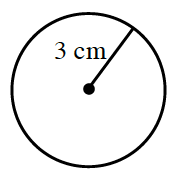b.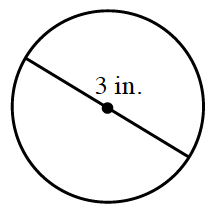c.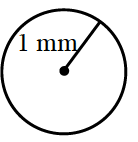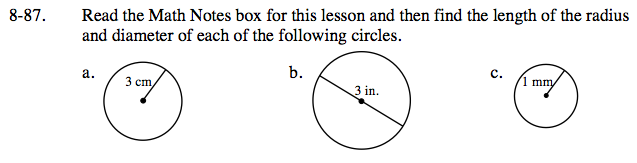Refer to the Math Notes box below in order to answer this question.